Courses

# Linear Algebra MCQ Level - 1

## 10 Questions MCQ Test Topic wise Tests for IIT JAM Physics | Linear Algebra MCQ Level - 1

Description
This mock test of Linear Algebra MCQ Level - 1 for IIT JAM helps you for every IIT JAM entrance exam. This contains 10 Multiple Choice Questions for IIT JAM Linear Algebra MCQ Level - 1 (mcq) to study with solutions a complete question bank. The solved questions answers in this Linear Algebra MCQ Level - 1 quiz give you a good mix of easy questions and tough questions. IIT JAM students definitely take this Linear Algebra MCQ Level - 1 exercise for a better result in the exam. You can find other Linear Algebra MCQ Level - 1 extra questions, long questions & short questions for IIT JAM on EduRev as well by searching above.
QUESTION: 1

### Let  A be a m × n matrix with row rank = r = column rank. The dimension of the space of solution of the system of linear equations AX = 0 is :

Solution:

Given that rank  A = r
⇒ There would be r  linearly independent solutions
Dim (A) = dim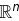– rank = n – r

The correct answer is: n – r

QUESTION: 2

### What would be the dimension for the general solution of the homogeneous system. x1 + 2x2 – 3x3 + 2x4 – 4x5 = 0 2x1 + 4x2 – 5x3 + x4 – 6x5 = 0 5x1 + 10x2 – 13x3 + 4x4 – 16x5 = 0

Solution:

Consider the coefficient matrix,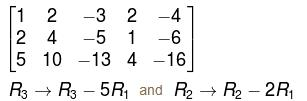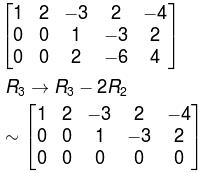The system in echelon form has three free variables,  x3x4x5
hence dim = 3

QUESTION: 3

### If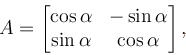then A-1 is equal to :

Solution: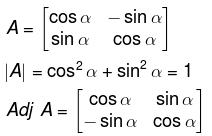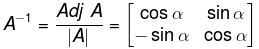The correct answer is: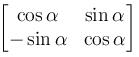QUESTION: 4

A matrix M has eigen values 1 and 4 with corresponding eigen vectors (1, –1)T  and  (2, 1)T, respectively. Then M  is :

Solution: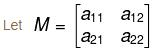We know that if λ is an eigenvalue of M, then X is the corresponding eigen vector then,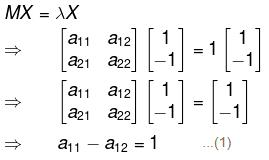a12 – a22 = –1       ...(2)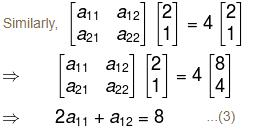2a21 + a22 = 4         ...(4)
Solving (1), (2), (3), (4), we get
a11 = 3, a12 = 2, a21 = 1, a22 = 2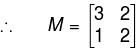The correct answer is: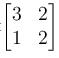QUESTION: 5

If rank of matrix A is 5 and nullity of A is 3, then A is of order :

Solution:

Rank is given by the number of non-zero rows the echelon from of the matrix and nullity is given by the Number of zero rows.

⇒   By sylvester's law, order of the matrix will be = rank + nullity
= 5 + 3
= 8

QUESTION: 6

The three equations,
–2x + y + z = a
x – 2y + z = b
x + y – 2z = c

will have no solution, unless :

Solution: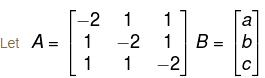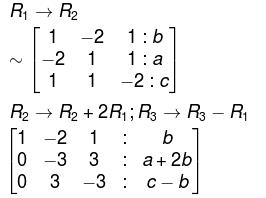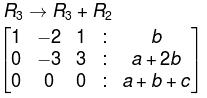Hence, the system won't contain any solution unless a + b + c becomes 0.

The correct answer is: a + b + c = 0

QUESTION: 7

Solving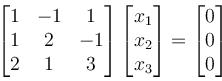will give,

Solution:

Consider the coefficient matrix, say  A, i.e.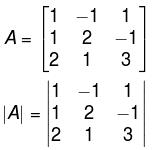= 1(6 + 1) + 1(3+2) + 1(1 – 4)

= 9 ≠ 0
Hence, rank  A = 3 = Number of unknowns.
∴ There will be only one solution of the given matrix equation and that is

x = y = z = 0.
The correct answer is: (0 0 0)T

QUESTION: 8

The matrix A is represented as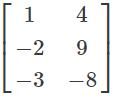. The transpose of the matrix of this matrix is represented as?

Solution:

Given matrix is a 3×2 matrix and the transpose of the matrix is 3 × 2 matrix. The values of matrix are not changed but the elements are interchanged, as row elements of a given matrix to the column elements of the transpose matrix and vice versa but the polarities of the elements remains same.

QUESTION: 9

Find the values of x, y, z and w from the below condition.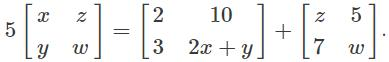Solution:

5z = 10 + 5 => 5z = 15 => z = 3
5x = 2 + z => 5x = 5 => x = 1
5y = 3 + 7 => 5y = 10 => y = 2
5w = 2 + 2 + w => 4w = 4 => w = 1.

QUESTION: 10

Let P be a matrix of order m × n and Q be a matrix of order n × p, n ≠ p. If rank (P) = n and rank of (Q) = p, then rank (PQ)  is :

Solution:

For the matrix P (m * n), rank<= min{ m,n}

Similarly for matrix Q (n * p), rank <= min{n , p}

Now, the rank of PQ <= min {rank of P ,rank of Q}

=> rank (PQ) <= min{ min {m,n} ,min{n,p}}

=> rank (PQ) <= min {m, n, p}